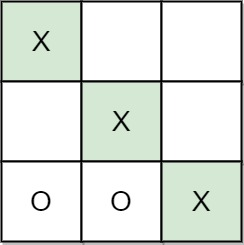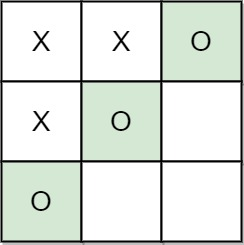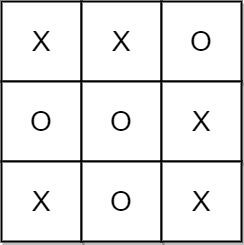1275. Find Winner on a Tic Tac Toe Game

#### QUESTION:

Tic-tac-toe is played by two players A and B on a 3 x 3 grid. The rules of Tic-Tac-Toe are:

Players take turns placing characters into empty squares ‘ ‘. The first player A always places ‘X’ characters, while the second player B always places ‘O’ characters. ‘X’ and ‘O’ characters are always placed into empty squares, never on filled ones. The game ends when there are three of the same (non-empty) character filling any row, column, or diagonal. The game also ends if all squares are non-empty. No more moves can be played if the game is over. Given a 2D integer array moves where moves[i] = [rowi, coli] indicates that the ith move will be played on grid[rowi][coli]. return the winner of the game if it exists (A or B). In case the game ends in a draw return “Draw”. If there are still movements to play return “Pending”.

You can assume that moves is valid (i.e., it follows the rules of Tic-Tac-Toe), the grid is initially empty, and A will play first.

Example 1:``````Input: moves = [[0,0],[2,0],[1,1],[2,1],[2,2]]
Output: "A"
Explanation: A wins, they always play first.
``````

Example 2:``````Input: moves = [[0,0],[1,1],[0,1],[0,2],[1,0],[2,0]]
Output: "B"
Explanation: B wins.
``````

Example 3:``````Input: moves = [[0,0],[1,1],[2,0],[1,0],[1,2],[2,1],[0,1],[0,2],[2,2]]
Output: "Draw"
Explanation: The game ends in a draw since there are no moves to make.
``````

__Constraints:__v

``````1 <= moves.length <= 9
moves[i].length == 2
0 <= rowi, coli <= 2
There are no repeated elements on moves.
moves follow the rules of tic tac toe.
``````

#### EXPLANATION:

1. 首先将棋子摆盘
2. 再进行判断, 分成横,竖和斜对角
3. 最后判断走的步数, 如果没到9说明是pending, 否则是draw

#### SOLUTION:

``````class Solution {
func tictactoe(_ moves: [[Int]]) -> String {
var board:[[String]] = [["","",""],["","",""],["","",""]]
var flag:Bool = true;
for move in moves {
var x:Int = move
var y:Int = move
if flag {
board[x][y] = "x"
} else {
board[x][y] = "o"
}
flag = !flag
}
// 处理横排
for i in board {
var pre:String = i
if pre != "" {
if pre == i && pre == i {
return getTictactoeResult(pre: pre)
}
}
}
// 处理竖排
for i in 0...2 {
var pre:String = board[i]
if pre != "" {
if pre == board[i] && pre == board[i] {
return getTictactoeResult(pre: pre)
}
}
}
// 处理斜对角
var pre = board
if pre != "" {
if pre == board && pre == board {
return getTictactoeResult(pre: pre)
}
}
pre = board
if pre != "" {
if pre == board && pre == board {
return getTictactoeResult(pre: pre)
}
}
if moves.count < 9 {
return "Pending"
} else {
return "Draw"
}
}

func getTictactoeResult(pre:String) -> String {
if pre == "x" {
return "A"
} else {
return "B"
}
return ""
}
}
``````